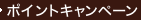•### Graphical Models in Applied Multivariate Statistics (Wiley Series in Probability and Mathematical Statistics. Probability and mathematical Statistics)

• 提携先の海外書籍取次会社に在庫がございます。通常2週間で発送いたします。
重要ご説明事項
1. 納期遅延や、ご入手不能となる場合が若干ございます。
2. 複数冊ご注文の場合、分割発送となる場合がございます。
3. 美品のご指定は承りかねます。
• ≪洋書のご注文につきまして≫ 「海外取次在庫あり」および「国内仕入れ先からお取り寄せいたします」表示の商品でも、納期の目安期間内にお届けできないことがございます。あらかじめご了承ください。

• 製本 Hardcover:ハードカバー版／ページ数 448 p.
• 言語 ENG
• 商品コード 9780471917502
• DDC分類 519.535

### Full Description

Graphical models----a subset of log--linear models----reveal the interrelationships between multiple variables and features of the underlying conditional independence. Following the theorem--proof--remarks format, this introduction to the use of graphical models in the description and modeling of multivariate systems covers conditional independence, several types of independence graphs, Gaussian models, issues in model selection, regression and decomposition. Many numerical examples and exercises with solutions are included.

### Table of Contents

`  Independence and Interaction.  Independence Graphs.  Information Divergence.  The Inverse Variance.  Graphical Gaussian Models.  Graphical Log-Linear Models.  Model Selection.  Methods for Sparse Tables.  Regression and Graphical Chain Models.  Models for Mixed Variables.  Decompositions and Decomposability.  Appendices.  References.  Author Index.  Subject Index.`